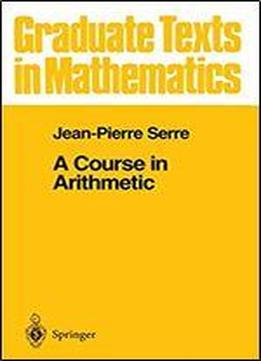# A Course In Arithmetic (graduate Texts In Mathematics, Vol. 7) by J-P. Serre / 1996 / English / PDF

This book is divided into two parts. The first one is purely algebraic. Its objective is the classification of quadratic forms over the field of rational numbers (Hasse-Minkowski theorem). It is achieved in Chapter IV. The first three chapters contain some preliminaries: quadratic reciprocity law, p-adic fields, Hilbert symbols. Chapter V applies the preceding results to integral quadratic forms of discriminant ± I. These forms occur in various questions: modular functions, differential topology, finite groups. The second part (Chapters VI and VII) uses "analytic" methods (holomor­ phic functions). Chapter VI gives the proof of the "theorem on arithmetic progressions" due to Dirichlet this theorem is used at a critical point in the first part (Chapter Ill, no. 2.2). Chapter VII deals with modular forms, and in particular, with theta functions. Some of the quadratic forms of Chapter V reappear here. The two parts correspond to lectures given in 1962 and 1964 to second year students at the Ecole Normale Superieure. A redaction of these lectures in the form of duplicated notes, was made by J.-J. Sansuc (Chapters I-IV) and J.-P. Ramis and G. Ruget (Chapters VI-VII). They were very useful to me I extend here my gratitude to their authors.

views: 185